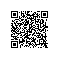# C++：运算符重载函数之"++"、"--"、"[ ]"、"=="的应用

5.2.5 "++"和"--"的重载

 对于前缀方式++ob，可以用运算符函数重载为：
ob.operator++()         //成员函数重载
或
operator++(X &ob)       //友元函数重载,其中ob为类X的对象的引用

对于后缀方式++ob，可以用运算符函数重载为：
ob.operator++(int)      //成员函数重载
或
operator++(X &ob,int)   //友元函数重载,其中ob为类X的对象的引用

调用时，参数int一般被传递给值0，例如：
class X{
...
public:
...
X operator++();         //前缀方式
X operator++(int);      //后缀方式
};
int main()
{
X ob;
...
++ob;                //隐式调用ob.operator++()
ob++;                //隐式调用ob.operator++(int)
ob.operator++();     //显式调用ob.operator++()，意为++ob
ob.operator++(0);    //显式调用ob.operator++(int),意为ob++
}

类似的，也可以重载为友元函数，例如：

class Y{
...
public:
...
firend Y operator++(Y &);         //前缀方式
friend Y operator++(Y &,int);      //后缀方式
};
int main()
{
Y ob;
...
++ob;                //隐式调用ob.operator++(Y&)
ob++;                //隐式调用ob.operator++(Y&,int)
operator++(ob);      //显式调用ob.operator++(Y&)，意为++ob
operator++(ob,0);    //显式调用ob.operator++(Y&,int),意为ob++
}

//例5.8 使用成员函数以前缀方式后后缀方式重载运算符"--"

#include<iostream>
using namespace std;
class Three{
public:
Three(int I1=0,int I2=0,int I3=0)
{
i1 = I1;
i2 = I2;
i3 = I3;
}
Three operator--();              //声明自减运算符--重载成员函数(前缀方式)
Three operator--(int a);        //声明自减运算符--重载成员函数(后缀方式)
void show();
private:
int i1,i2,i3;
};
Three Three::operator--()           //定义自减运算符--重载成员函数(前缀方式)
{
--i1;
--i2;
--i3;
return *this;   //返回自减后的当前对象
}
Three Three::operator--(int a)          //定义自减运算符--重载成员函数(后缀方式)
{
Three temp(*this);
i1--;
i2--;
i3--;
return temp;  //返回自减前的当前对象
}
void Three::show()
{
cout<<"i1="<<i1<<",";
cout<<"i2="<<i2<<",";
cout<<"i3="<<i3<<endl;
}
int main()
{
Three t1(4,5,6),t2,t3(11,12,13),t4;
t1.show();

--t1;                       //隐式调用(前缀方式)
t1.show();                  //显示执行--t1后的值
t2=t1--;                      //隐式调用(后缀方式)，将t1自减前的值赋给t2
t2.show();                  //显示t2保存的是执行t1--之前的t1的值
t1.show();                  //显示执行t1--之后的t1的值
cout<<endl;

t3.show();
t3.operator--();           //显示调用(前缀方式)
t3.show();
t4=t3.operator--(0);       //显示调用(后缀方式)
t4.show();
t3.show();

return 0;
}

//例5.8 使用友元函数以前缀方式和后缀方式重载运算符"++"

#include<iostream>
using namespace std;
class Three{
public:
Three(int I1=0,int I2=0,int I3=0)
{
i1 = I1;
i2 = I2;
i3 = I3;
}
friend Three operator++(Three &);              //声明自加运算符++重载成员函数(前缀方式)
friend Three operator++(Three &,int );         //声明自加运算符++重载成员函数(后缀方式)
void show();
private:
int i1,i2,i3;
};
Three operator++(Three &T)                //声明自加运算符++重载友元函数(前缀方式)
{
++T.i1;
++T.i2;
++T.i3;
return T;   //返回自加后的对象
}
Three operator++(Three &T,int a)          //声明自加运算符--重载友元函数(后缀方式)
{
Three temp(T);
T.i1++;
T.i2++;
T.i3++;
return temp;  //返回自加前的对象
}
void Three::show()
{
cout<<"i1="<<i1<<",";
cout<<"i2="<<i2<<",";
cout<<"i3="<<i3<<endl;
}
int main()
{
Three t1(4,5,6),t2,t3(14,15,16),t4;
t1.show();

++t1;                       //隐式调用(前缀方式)
t1.show();                  //显示执行++t1后的值
t2=t1++;                      //隐式调用(后缀方式)，将t1自加前的值赋给t2
t2.show();                  //显示t2保存的是执行t1++之前的t1的值
t1.show();                  //显示执行t1++之后的t1的值
cout<<endl;

t3.show();
operator++(t3);           //显示调用(前缀方式)
t3.show();
t4=operator++(t3,0);       //显示调用(后缀方式)
t4.show();
t3.show();

return 0;
}

（1）由于友元运算符重载函数没有this指针，所以不能引用this指针所指的对象，使用友元函数重载增运算符"++"或自减"--"时，应采用对象引用传递数据。

friend Three operator++(Three &); //声明自加运算符++重载成员函数(前缀方式)
friend Three operator++(Three &,int ); //声明自加运算符++重载成员函数(后缀方式)

（2）前缀方式和后缀方式的函数内部语句可以相同，也可以不同，取决于编程的需要。

5.2.7 下标运算符"[]"的重载

[]---------双目运算符
X----------左操作数
Y----------右操作数

X[Y]   被解释为    X.operator[](y);

//函数体
｝

//例5.12 使用下标运算符重载函数的引例

#include<iostream>
using namespace std;
class Vector4{
public:
Vector4(int a1,int a2,int a3,int a4)
{
v=a1;
v=a2;
v=a3;
v=a4;
}
private:
int v;
};
int main()
{
Vector4 ve(1,2,3,4);
cout<<v;   //运行错误，v是类Vector4的私有成员，即使是公有成员，输出格式应为ve.v;
return 0;
}

可是，如果对[]进行重载，即使v是私有成员，也可以运行成功，直接访问。即：
int &Vector::operator[](int bi)
{
if(bi<0||bi>=4)
{
cout<<"Bad subscript!\n";
exit(1);
}
return v[bi];  //v[bi]被解释为v.operator[](2)
}

//例5.13 使用下标运算符[]重载函数

#include<iostream>
using namespace std;
class Vector4{
public:
Vector4(int a1,int a2,int a3,int a4)
{
v=a1;
v=a2;
v=a3;
v=a4;
}
int &operator[](int );
private:
int v;
};
int &Vector4::operator[](int bi)  //返回一个int型的引用
{
if(bi<0||bi>=4)
{
cout<<"Bad subscript!\n";
exit(1);
}
return v[bi];  //v[bi]被解释为v.operator[](2)
}
int main()
{
Vector4 v(1,2,3,4);
int i=0;
for(;i<4;i++)
cout<<"v["<<i<<"]="<<v[i]<<"\n";  //v[i]被解释为v.operator[](i);
cout<<endl;

v=v;        //v被解释为v.operator[](2);
cout<<"v="<<v<<endl; //v被解释为v.operator[](3);

v=22;
cout<<"v="<<v<<endl;

return 0;
}
/*

v=1
v=2
v=3
v=4

v=3
v=22
*/

5.2.6 赋值运算符"="的重载

X &X::operator=(const X &source)
{
//成员间赋值

obj1=obj2;

1.指针悬挂问题

//例 5.10 关于浅层复制的例子。

#include<iostream>
using namespace std;
class STRING{
public:
STRING(char* s)
{
cout<<"Constructor called."<<endl;
pt = new char(strlen(s)+1);
strcpy(pt,s);
}
~STRING()
{
cout<<"Destructor called."<<pt<<endl;
delete pt;
}
private:
char* pt;
};
int main()
{
STRING p1("book");
STRING p2("jeep");
p2=p1;
return 0;
}

Constructor called. (1)
Constructor called. (2)
Destructor called.book (3)
Destructor called.*q (4)

2.用深层复制解决指针悬挂问题

//例5.11 关于深层赋值的例子

#include<iostream>
using namespace std;
class STRING{
public:
STRING(char* s)
{
cout<<"Constructor called."<<endl;
pt = new char(strlen(s)+1);
strcpy(pt,s);
}
STRING &operator=(const STRING &s);     //声明赋值运算符重载函数
~STRING()
{
cout<<"Destructor called."<<pt<<endl;
delete pt;
}
private:
char* pt;
};
STRING &STRING::operator=(const STRING &s) //定义赋值运算符重载函数
{
if(this==&s)  return *this;         //防止s=s的赋值
delete pt;                          //释放掉原区域
pt = new char(strlen(s.pt)+1);      //分配新区域
strcpy(pt,s.pt);                    //字符串复制
return *this;
}
int main()
{
STRING p1("book");
STRING p2("jeep");
p2=p1;
return 0;
}

Constructor called.
Constructor called.
Destructor called.book
Destructor called.book

friend string &operator=(string &p2,string &p1)

p1="book" 将被解释为 operator=(p1,book) 这显然是没有问题的，使用钉钉扫一扫加入圈子
+ 订阅